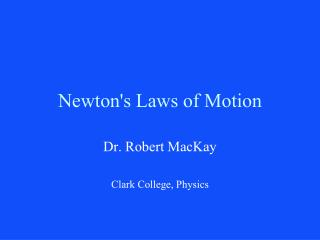DownloadDownload PresentationNewton's Laws of Motion

# Newton's Laws of Motion

Télécharger la présentation## Newton's Laws of Motion

- - - - - - - - - - - - - - - - - - - - - - - - - - - E N D - - - - - - - - - - - - - - - - - - - - - - - - - - -
##### Presentation Transcript

1. Newton's Laws of Motion Dr. Robert MacKay Clark College, Physics

2. Introduction • Newtons 3 laws of motion • 1. Law of inertia • 2. Net Force = mass x acceleration • ( F = M A ) • 3. Action Reaction • Newton’s Universal Law of Gravity Newton came up with these laws in about 1670 to describe the motion of the planets around the sun. These fairly simple rules explained planetary motion extremely well. This was a problem scientists had struggled with for thousands of years with no success. These same laws apply to anything that moves including the atmosphere and winds.

3. Isaac Newton 1642-1727 Isaac Newton 1689 Knighted by Queen Anne 1705 Isaac Newton 1702 Isaac Newton 1726

4. To understand Newton’s laws of motion one must first understand velocity. • The velocity of an object is related to how fast an object is moving and in what direction. Your velocity changes any time that your speed changes or your direction changes (or both).

5. Next we need to understand what we mean by acceleration. • The acceleration of an object describes how fast its velocity changes. • If an object travels in a straight line with constant speed the acceleration is zero. • Whenever an object either changes speed of changes direction it is accelerating. • In the metric system acceleration is expressed in term of meters per second (m/s) increase over each second (s). For example is a car speeds up from 0 to 20 m/s speed in 4.0 s its acceleration is 5.0 m/s each second (5 m/s2)

6. Law of inertia (Newton’s 1st Law) • Every object continues in its state of rest, or of uniform motion in a straight line, unless it is compelled to change that state by forces impressed upon it. • acceleration = 0.0 unless the objected is acted on by an unbalanced force • Example: When the Coriolis force exactly balance the pressure gradient force the wind travels in a straight path at constant speed.

7. Newton’s 2nd Law • Net Force = Mass x Acceleration • Net_F = M A • The metric system unit for Force is a Newton (N). A force of 1.0 N is required to accelerate a 1.0 kg object at a rate of 1.0 m/s2.

8. Newton’s 2nd Law • Net Force = Mass x Acceleration • F = M A M=2.0 kg F=? F=M A = 2.0 kg x 6.0 m/s2 =12.0 Newtons = 12.0 N A= 6.0 m/s2

9. An object experiences a net force and exhibits an acceleration in response. Which of the following statements is always true? (a) The object moves in the direction of the force. (b) The acceleration is in the same direction as the velocity. (c) The acceleration is in the same direction as the force. (d) The velocity of the object increases.

10. An object experiences a net force and exhibits an acceleration in response. Which of the following statements is always true? (a) The object moves in the direction of the force. (b) The acceleration is in the same direction as the velocity. X(c) The acceleration is in the same direction as the force. (d) The velocity of the object increases.

11. Newton’s 2nd Law • Net Force = Mass x Acceleration • F = M A M=2.0 kg F=6.0 N A=F / M = ? m/s2 A= ?

12. Newton’s 2nd Law • Net Force = Mass x Acceleration • F = M A M=2.0 kg F=6.0 N A=F / M = 3.0 m/s2 A= 3.0 m/s2

13. Newton’s 2nd Law • Net Force = Mass x Acceleration • F = M A M=? F= 10.0 N M= F/A = ? kg A= 20.0 m/s2

14. Newton’s 2nd Law • Net Force = Mass x Acceleration • F = M A M=? F= 10.0 N M= F/A = 0.50 kg A= 20.0 m/s2

15. Newton’s 2nd Law • Net Force = Mass x Acceleration • F = M A F= 150.0 N M= 5.0 kg D =120.0 N Net Force = ? A= ? m/s2

16. Newton’s 2nd Law • Net Force = Mass x Acceleration • F = M A F= 150.0 N M= 5.0 kg D =120.0 N 1. Net Force = 30.0 N 2. A= 6.0 m/s2